# Quantum Grassmannian

(diff) ← Older revision | Latest revision (diff) | Newer revision → (diff)

A subalgebrain the algebraof regular functions on the quantum group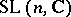(cf. Quantum groups).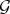is generated by quantum minors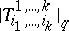, with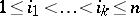and with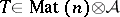the vector co-representation of[a1]. The-minors satisfy quadratic relations, which turn into the Plücker relations (Young symmetries, cf. also Grassmann manifold) when the deformation parameteris specialized to. Since classically the Grassmannian, as a complex submanifold in the projective space, is the common zero locus of the Plücker relations, one interpretsas a quantization of the complex Poisson manifold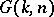(cf. Symplectic structure). The co-multiplicationininduces a right co-action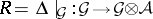and sois a quantum homogeneous space.

A more general construction of (generalized) quantum flag manifolds exists for the group[a1], as well as for other simple complex Lie groupshaving quantum counterparts [a2]. Another description was given in [a3]. Both approaches [a2], [a3] also allow one to define quantum Schubert varieties.

Sinceis compact, the only holomorphic functions defined globally on it are the constants. But one can work instead with holomorphic coordinates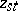,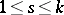,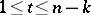, on the big cell, the unique Schubert cell of top dimension. The standard choice of coordinates is given via the Gauss decomposition of. For the algebrathis means in fact a localization by allowing the-minor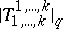to be invertible. The generatorsof the quantum big cell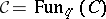satisfy the relations [a4]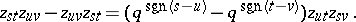The symplectic manifoldcan be realized as an orbit of the dressing transformation of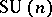acting on its dual Poisson Lie group. The transformation can be also viewed as the right-action on the manifoldof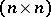unimodular positive matrices: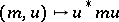. The orbits are determined by sets of eigenvalues andcorresponds to a two-point set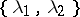with multiplicitiesand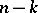, respectively. There exists a quantum analogue as a right co-action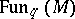is endowed with a-involution and, correspondingly, one can turninto a-algebra by determining the commutation relations betweenand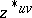in dependence on the parameters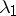and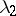[a4].

Similarly as for quantum spheres (cf. Quantum sphere), other types of quantum Grassmannians have been defined, distinguished by possessing classical points, i.e., one-dimensional representations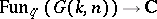[a5].

How to Cite This Entry:
Quantum Grassmannian. Encyclopedia of Mathematics. URL: http://encyclopediaofmath.org/index.php?title=Quantum_Grassmannian&oldid=15744
This article was adapted from an original article by Pavel Å tovÃ­Äek (originator), which appeared in Encyclopedia of Mathematics - ISBN 1402006098. See original article